1.2 Introducing the cosmological constant

Einstein's original field equations are(1)

(I use conventions in which c = 1, and will also set= 1 in most of the formulae to follow, but Newton's constant will be kept explicit.) On very large scales the universe is spatially homogeneous and isotropic to an excellent approximation, which implies that its metric takes the Robertson-Walker form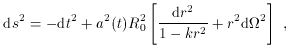(2)

where d2 = d2 + sin2d2 is the metric on a two-sphere. The curvature parameter k takes on values +1, 0, or -1 for positively curved, flat, and negatively curved spatial sections, respectively. The scale factor characterizes the relative size of the spatial sections as a function of time; we have written it in a normalized form a(t) = R(t) / R0, where the subscript 0 will always refer to a quantity evaluated at the present time. The redshift z undergone by radiation from a comoving object as it travels to us today is related to the scale factor at which it was emitted by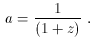(3)

The energy-momentum sources may be modeled as a perfect fluid, specified by an energy densityand isotropic pressure p in its rest frame. The energy-momentum tensor of such a fluid is(4)

where Uµ is the fluid four-velocity. To obtain a Robertson-Walker solution to Einstein's equations, the rest frame of the fluid must be that of a comoving observer in the metric (2); in that case, Einstein's equations reduce to the two Friedmann equations(5)

where we have introduced the Hubble parameter H/ a, and(6)

Einstein was interested in finding static (= 0) solutions, both due to his hope that general relativity would embody Mach's principle that matter determines inertia, and simply to account for the astronomical data as they were understood at the time. (1) A static universe with a positive energy density is compatible with (5) if the spatial curvature is positive (k = +1) and the density is appropriately tuned; however, (6) implies thatwill never vanish in such a spacetime if the pressure p is also nonnegative (which is true for most forms of matter, and certainly for ordinary sources such as stars and gas). Einstein therefore proposed a modification of his equations, to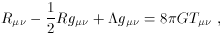(7)

where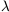is a new free parameter, the cosmological constant. Indeed, the left-hand side of (7) is the most general local, coordinate-invariant, divergenceless, symmetric, two-index tensor we can construct solely from the metric and its first and second derivatives. With this modification, the Friedmann equations become(8)

and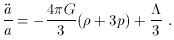(9)

These equations admit a static solution with positive spatial curvature and all the parameters, p, andnonnegative. This solution is called the ``Einstein static universe."

The discovery by Hubble that the universe is expanding eliminated the empirical need for a static world model (although the Einstein static universe continues to thrive in the toolboxes of theorists, as a crucial step in the construction of conformal diagrams). It has also been criticized on the grounds that any small deviation from a perfect balance between the terms in (9) will rapidly grow into a runaway departure from the static solution.

Pandora's box, however, is not so easily closed. The disappearance of the original motivation for introducing the cosmological constant did not change its status as a legitimate addition to the gravitational field equations, or as a parameter to be constrained by observation. The only way to purgefrom cosmological discourse would be to measure all of the other terms in (8) to sufficient precision to be able to conclude that the/3 term is negligibly small in comparison, a feat which has to date been out of reach. As discussed below, there is better reason than ever before to believe thatis actually nonzero, and Einstein may not have blundered after all.

1 This account gives short shrift to the details of what actually happened; for historical background see . Back.